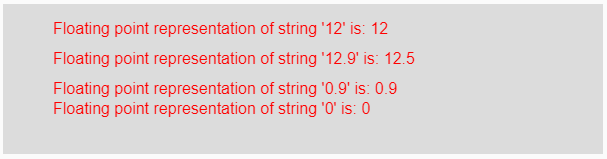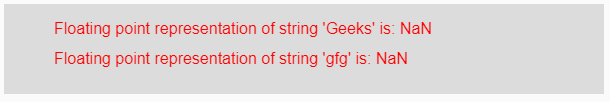# p5.js | float() function

The float() function in p5.js is used to get the floating point representation of the given string as a parameter.

Syntax:

`float(String)`

Parameters: This function accepts a single parameter String which is used to be converted into its floating point representation.

Return Value: It returns the converted floating point representation.

Below programs illustrate the float() function in p5.js:

Example 1: This example uses float() function to get the floating point representation of the given string as a parameter.

 `function` `setup() {  ` `  `  `    ``// Creating Canvas size ` `    ``createCanvas(600, 150);  ` `}  ` `  `  `function` `draw() {  ` `      `  `    ``// Set the background color  ` `    ``background(220);  ` `    `  `    ``// Initializing some strings ` `    ``let String1 = ``"12"``; ` `    ``let String2 = ``"12.5"``; ` `    ``let String3 = ``"0.9"``; ` `    ``let String4 = ``"0"``; ` `    `  `    ``// Calling to float() function. ` `    ``let A = float(String1); ` `    ``let B = float(String2); ` `    ``let C = float(String3); ` `    ``let D = float(String4); ` `      `  `    ``// Set the size of text  ` `    ``textSize(16);  ` `      `  `    ``// Set the text color  ` `    ``fill(color(``'red'``));  ` `    `  `    ``// Getting floating point representation ` `    ``text(``"Floating point representation of string '12' is: "` `+ A, 50, 30); ` `    ``text(``"Floating point representation of string '12.9' is: "` `+ B, 50, 60); ` `    ``text(``"Floating point representation of string '0.9' is: "` `+ C, 50, 90); ` `    ``text(``"Floating point representation of string '0' is: "` `+ D, 50, 110); ` `}   `

Output:Example 2: This example uses float() function to get the floating point representation of the given string as a parameter.

 `function` `setup() {  ` `  `  `    ``// Creating Canvas size ` `    ``createCanvas(600, 90);  ` `}  ` `  `  `function` `draw() {  ` `      `  `    ``// Set the background color  ` `    ``background(220);  ` `    `  `    ``// Initializing some strings ` `    ``let String1 = ``"Geeks"``; ` `    ``let String2 = ``"gfg"``; ` `    `  `    ``// Calling to float() function. ` `    ``let A = float(String1); ` `    ``let B = float(String2); ` `      `  `    ``// Set the size of text  ` `    ``textSize(16);  ` `      `  `    ``// Set the text color  ` `    ``fill(color(``'red'``));  ` `    `  `    ``// Getting floating point representation ` `    ``text(``"Floating point representation of string 'Geeks' is: "` `            ``+ A, 50, 30); ` `    ``text(``"Floating point representation of string 'gfg' is: "`  `            ``+ B, 50, 60); ` `}  `

Output:Note: From the above example, if the parameter should be a number then it returns the output as floating point representation otherwise it returns NaN i.e, not a number.

Reference: https://p5js.org/reference/#/p5/float

My Personal Notes arrow_drop_upCheck out this Author's contributed articles.

If you like GeeksforGeeks and would like to contribute, you can also write an article using contribute.geeksforgeeks.org or mail your article to contribute@geeksforgeeks.org. See your article appearing on the GeeksforGeeks main page and help other Geeks.

Please Improve this article if you find anything incorrect by clicking on the "Improve Article" button below.

Article Tags :

Be the First to upvote.

Please write to us at contribute@geeksforgeeks.org to report any issue with the above content.